Tags
#cfa #economics #has-images
Question

Mathematically, the inverse relationship of the Law of Demand may be expressed as a causal relation: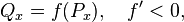where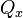is the quantity demanded of good x,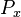is the price of the good,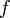is the demand function, and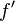is its derivative.

Here,is the [another name for the independent variable?] (independent variable) andIs the dependent variable

causal factor (i.e. the "cause")

Tags
#cfa #economics #has-images
Question

Mathematically, the inverse relationship of the Law of Demand may be expressed as a causal relation:whereis the quantity demanded of good x,is the price of the good,is the demand function, andis its derivative.

Here,is the [another name for the independent variable?] (independent variable) andIs the dependent variable

?

Tags
#cfa #economics #has-images
Question

Mathematically, the inverse relationship of the Law of Demand may be expressed as a causal relation:whereis the quantity demanded of good x,is the price of the good,is the demand function, andis its derivative.

Here,is the [another name for the independent variable?] (independent variable) andIs the dependent variable

causal factor (i.e. the "cause")
If you want to change selection, open original toplevel document below and click on "Move attachment"

#### Parent (intermediate) annotation

Open it
inverse relationship of the Law of Demand may be expressed as a causal relation: where is the quantity demanded of good x, is the price of the good, is the demand function, and is its derivative. Here, is the <span>causal factor (independent variable) and Is the dependent variable <span><body><html>

#### Original toplevel document

Law of demand - Wikipedia, the free encyclopedia
ction 3 Exceptions to the law of demand 3.1 Giffen goods 3.2 Expectation of change in the price of commodity 3.3 Basic or necessary goods 4 The law of demand and change in demand 5 See also 6 References Mathematical expression <span>Mathematically, the inverse relationship may be expressed as a causal relation: where is the quantity demanded of good x, is the price of the good, is the demand function, and is its derivative. Here, is the causal factor (independent variable) and Is the dependent variable. Graphical depiction A demand curve is a graphical depiction that abides by the law of demand. It shows how the quantity demanded of some product during a specified period of

#### Summary

status measured difficulty not learned 37% [default] 0

No repetitions